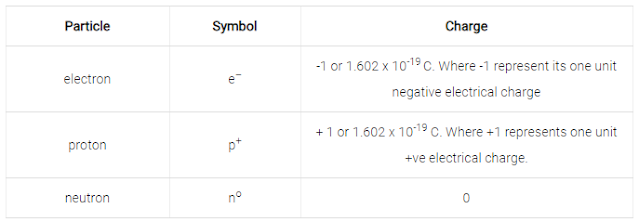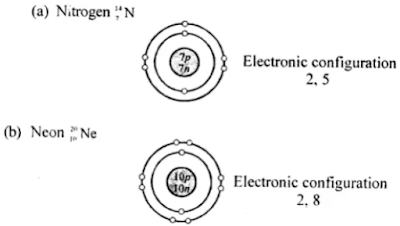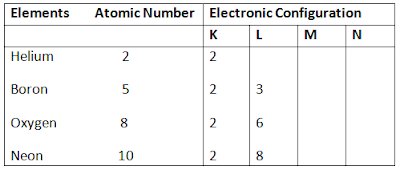# Selina Concise Solutions for Chapter 4 Atomic Structure Class 8 Chemistry ICSE

##### Exercise

1. Fill in the blanks.
(a) Dalton         that atoms could not be divided
(b) An ion which has a positive charge is called a         .
(c) The outermost shell of an atom is known as           .
(d) The           of an atom is very hard and dense.
(e) Neutrons are neutral particles having mass equal to that of protons.
(f) Isotopes are the atoms of             having the same atomic number but a different mass number.
(a) Dalton said that atoms could not be divided
(b) An ion which has a positive charge is called a cation.
(c) The outermost shell of an atom is known as valence shell.
(d) The nucleus of an atom is very hard and dense.
(e) Neutrons are neutral particles having mass equal to that of protons.
(f) Isotopes are the atoms of an element having the same atomic number but a different mass number.

2. Write ‘true’ or ‘false’ for the following statements:

(a) An atom on the whole has a positive charge.
false

(b) The maximum number of electrons in the first shell can be 8.
false

(c) The central pad of the atom is called nucleus.
True.

3. Give the following a suitable word/phrase.
(a) The sub-atomic particle with negative charge and negligible mass.
(b) Protons and neutrons present in the nucleus.
(c) The electrons present in the outermost shell.
(d) Arrangement of electrons in the shells of an atom.
(e) The number of protons present in the nucleus of an atom.
(f) The sum of the number of protons and neutrons of an atom.
(g) Atoms of same element with same atomic number but a different mass number.
(h) The smallest unit of an element which takes part in a chemical reaction.
(a) Neutron
(b) Mass number
(c) Valency
(d) Orbits or Valence shells
(e) Atomic number
(f) Mass number
(g) Isotopes
(h) Atom

4. Multiple Choice Questions

(a) The outermost shell of an atom is known as
1. valency
2. valence electrons
3. nucleus
4. valence shell
2. valence electrons

(b) The number of valence electrons present in magnesium is
1. two
2. three
3. four
4. five
1. two

(c) The sub atomic particle with negative charge is
1. proton
2. neutron
3. electron
4. nucleon
3. electron

(d) If the atomic number of an atom is 17 and mass number is 35 then number of neutron will be
1. 35
2. 17
3. 18
4. 52
3. 18

(e) The number of electrons in an atom is equal to number of
1. protons in a neutral atom
2. neutrons in a neutral atom
3. nucleons in a neutral atom
4. none of the above
1. protons in a neutral atom

(f) The sum of number of protons and number of neutrons present in the nucleus of an atom is called its
1. mass number
2. atomic number
3. number of electrons
4. all of the above
1. mass number

5. Name three fundamental particles of the atom. Give the symbol with charge, on each particle.
The fundamental particles of the atom are: electrons, protons and neutrons.6. Define the following terms:
(a) Atomic number
(b) Mass number
(c) Nucleons
(d) Valence shell
(a) Atomic number: Atomic number refers to the number of protons present in an atom. It is denoted by Z. Example: An atom of oxygen contains 8 proton Therefore its atomic number is 8.

(b) Mass number: Mass number refers to the sum of the number of protons and neutrons present in the nucleus of an atom and denoted by A Mass number = Number of protons + Number of neutrons.

(c) Nucleons: The protons and neutrons collectively are known as nucleons.

(d) Valence Shell: The outermost shell of an atom is known as its valence shell.

7. Mention briefly the salient features of Dalton’s atomic theory (five points).
Salient features of Dalton’s atomic theory:
1. Matter consists of very small and indivisible particles called atoms, which can neither be created nor can be destroyed.
2. The atoms of an element are alike in all respects i.e. size, mass, density, chemical properties but they differ from the atoms of other elements.
3. Atoms of an element combine in small numbers to form molecules of the element.
4. Atoms of one element combine with atoms of another element in simple whole number ratio to form molecules of compounds.
5. Atoms are the smallest units of matter that can take part in a chemical reaction during which only rearrangement of atoms takes place.

8. (a) What are the two main features of Rutherford’s atomic model?
(b) State its one drawback.
(a) According to Rutherford’s model an atom consists of:
1. The centrally located nucleus: The nucleus is a centrally located positively charged mass. The entire mass of the atom is concentrated in it. It is the densest part of the atom. Its size is very small as compare to the atom as a whole.
2. The outer circular orbits: Electrons revolve in circular orbits (shell) in the space available around the nucleus. An atom is electrically neutral i.e., number of protons and electrons present in an atom are equal.

(b) Rutherford’s atomic model could not explain the stability of the atom as it is like a solar system, the sun is at the centre and the planets revolve around it, in an atom the electrons revolve around the centrally located nucleus containing protons.

9. What are the observations of the experiment done by Rutherford in order to determine the structure of an atom?
Following were the observations made by Rutherford:
1. Most of the alpha particle passed straight through the foil without any deflection from their path.
2. A small fraction of them were deflected from their original path by small angles.
3. Only a few particles bounced back.

10. State the mass number, the atomic number, number of neutrons and electronic configuration of the following atoms.Also, draw atomic diagrams for them.11. What is variable valency? Name two elements having variable valency and state their valencies.
Variable valency: Some elements exhibit more than one valency. They are said to have variable valency, e.g. Iron, copper, tin, lead.

12. The atomic number and the mass number of sodium are 11 and 23 respectively. What information is conveyed by this statement.
Atomic number = 11;   No of protons = 11
Mass number = 23 = Number of protons + Number of neutrons.
No of neutrons = 23 - 11 = 12.

13. Draw the diagrams representing the atomic structures of the following:
(a) Nitrogen (b) Neon14. Explain the rule with example according to which electrons are filled in various energy levels,
The maximum number of electrons that can be present in any shell or orbit of an atom is given by the formula 2n2, where n is the serial number of the shell.
Therefore:
K shell, n = 1, no. of electrons = 2×12 = 2
L shell, n = 2, no. of electrons = 2×22 = 8
M shell, n = 3, no. of electrons = 2×32 = 18
N shell, n = 4, no. of electrons = 2×42 = 32
Electrons are not accommodated in a given shell, unless the inner shells are filled.
That is, the shells are filled in a stepwise manner.
Example:15. The atom of an element is made up of 4 protons, 5 neutrons and 4 electrons. What is its atomic number and mass number?
Protons = 4, neutrons = 5, electrons = 4
Atomic number = 4,
Mass number = 4 + 5 = 9

16. (a) What are the two main parts of which an atom is made of?
(b) Where is the nucleus of an atom situated?
(c) What are orbits or shells of an atom?
(a) 1. The centrally located nucleus
2. The outer circular orbits.
(b) The nucleus is a centrally located positively charged mass.
(c) The circular orbits (shell present) in the space available around the nucleus on which electrons revolve are called orbits or shells of an atom.

17. What are isotopes? How does the existence of isotopes contradict Dalton’s atomic theory?
Atoms of an element must have the same atomic number, but their mass number can be different due to the presence of different number of neutrons. These atoms of an element having different number of neutrons are called groups.
According to Dalton’s theory, all atoms of an element are similar to all respects, for example, they have the same shape, size etc. and have similar physical and chemical properties like mass, density and reactivity. Whereas isotopes of an element have atoms that are similar as they have same number of protons and electrons but differ in the number of neutrons. So, the isotopes have atoms that are not similar in all aspects.

18. Complete the table below by identifying A, B, C, D, E and F.### Extra Practice Based Questions

Fill in the Blanks

1. Atomic theory of matter was given by             .
2. Gases become good conductors of electricity under       (low/high) pressure and        (low/high) voltage electrical discharge.
3. Rutherford won the Nobel Prize in 1908 for his investigations in the field of           .
4. The electrons revolving in shells close to the nucleus are called          electrons.
5. In an atom, the last shell containing electrons is called its           shell.
6. The protons and neutrons are held firmly in the nucleus by strong          .
7. Amount of energy stored inside the          is known as nuclear energy.
8. The γ-rays are                radiations, like light.
9. Nuclear energy can be used to make
1. Atomic theory of matter was given by Democritus.
2. Gases become good conductors of electricity under low (low/high) pressure and high (low/high) voltage electrical discharge.
3. Rutherford won the Nobel Prize in 1908 for his investigations in the field of atomic theory.
4. The electrons revolving in shells close to the nucleus are called bound electrons.
5. In an atom, the last shell containing electrons is called its valence shell.
6. The protons and neutrons are held firmly in the nucleus by strong nuclear forces.
7. Amount of energy stored inside the nucleus is known as nuclear energy.
8. The γ-rays are electromagnetic radiations, like light.
9. Nuclear energy can be used to make electricity.

### Multiple Choice Questions

1. Which of these have similar chemical properties but different set of physical properties and mass number?
1. isobars
2. elements
3. isotopes
4. none of these
3. isotopes

2. Which one of the following scientists put forward the theory regarding the extra-nuclear structure of the atom?
2. John Dalton
3. Rutherford
4. Niels Bohr
4. Niels Bohr

3. Which of the following scientists observed that cathode rays consists of negatively charged particles?
1. John Dalton
2. J J Thomson
4. Democritus
2. J J Thomson

4. In 1908, R A Millikan found the charge of an electron to be
1. 1.6×10-19 C
2. 1.6×10-15 C
3. 1.4×10-19 C
4. 1.6×10-16 C.
1. 1.6×10-19 C

5. How many times is a proton heavier than an electron?
1. 1500
2. 1800
3. 1840
4. none of these
3. 1840

6. Who discovered that radioactive rays are given out by uranium?
1. Henri Becquerel
2. Marie Curie
3. Pierre Curie
4. Schmidt
2. Marie Curie

7. β-particles are actually a stream of
1. protons
2. electrons
3. neutrons
4. none of these
2. electrons

8. Which of the following is used as a coolant in nuclear reactor?
1. liquid sodium
2. liquid nitrogen
4. plutonium
1. liquid sodium

1. Elements combine in definite proportions to form compounds.
True.

2. Elements having a tendency to accept electrons are called metals.
False.
Element having a tendency to release/donate electrons are called metals.

3. Electrons are present in the nucleus.
False.
Electrons are present in the shells.

4. Neils Bohr gave the theory to explain the arrangement of protons in an atom.
False.
Neils Bohr gave the theory to explain the arrangement of electrons in an atom.

5. Cathode rays are a stream of fast moving particles that travel in random directions.
False.
Cathode rays are a stream of fast moving particles that travel in straight line.

6. Uranium-235 and uranium-238 both undergo nuclear fission.
True.

7. After the emission of a beta-particle, the atomic number of the atom increases by one.
True.

1. What is an atom?
An atom is defined as the smallest particle of a molecule which is further subdivided into electrons, protons and neutrons.

2. Describe a discharge tube.

A discharge tube is a cylindrical glass tube in which a gas can be filled and which is fitted with metal plates called electrodes at each end.

3. What is the central part of an atom known as?

Nucleus consisting of neutrons and protons.

4. What do you mean by electron transition?

The jumping of an electron from one energy level to another is called electronic transition.

5. Define an ionic compound.
An ionic compound is defined as the compound formed by the oppositely charged ions which attract each other to form a chemical bond e.g. Sodium chloride.

6. Define the atomic mass number of an atom.

The atomic mass number refers to total number of protons and neutrons in a nucleus.

7. Name the isotopes of hydrogen.

There are 3 (three) isotopes of hydrogen
1. Protium 1H1
2. Deuterium 1H2
3. Tritium 1H3

9. Name three inert gases.

Inert gas: Argon, Helium, Neon.

10. What is the charge on a neutron ?

No charge.

11. What is the number of neutrons present in a potassium atom ?

20

1. State the postulates of the Dalton’s atomic theory.

Postulates of Dalton’s atomic theory:
1. All matter is made up of minute particles called atoms.
2. 2. Atom are indivisible. They can neither be created nor destroyed.
3. 3. All atoms of a given element are identical in mass and size.
4. 4. The atoms of one element are different from the atoms of other elements.
5. 5. During a chemical reaction, the atoms of an element combine with atoms of another element in a fixed ratio.

2. Name the three subatomic particles. How are they different from each other ?

The three subatomic particles are:
1. Electron: It is represented by e it has a unit negative charge and possesses mass equal to 1/1837 times that of a proton.
2. Proton: It is represented by +1p1. It has a unit positive charge. Its mass is equal to the mass of a hydrogen atom.
3. Neutron: It is denoted as 0n1. It has no charge on it. Its mass is almost equal to the mass of hydrogen atom.

3. Enlist the features of Bohr’s model of an atom. How did he correct the Rutherford’s model ?

Bohr’s Postulates:
1. 1. The extra-nuclear region of an atom consists of electrons revolving in particular orbits called shells.
2. 2. Each shell has a fixed energy level.
3. 3. As long as an electron revolves in the same shell, the energy remains constant.
During electronic transition energy is either released or absorbed.
Bohr corrected Rutherford’s model by suggesting the concepts of energy levels and electronic transition.

4. What is the maximum number of electrons that L shell can accommodate ? Give reasons for your answer.

The maximum possible number of electrons that a shell can accommodate can be given by the formula 2nr.
(Here n is the number of shell)
In case of L shell n = 2
∴ 2n2
= 2(2)2
= 8
Thus L shell can accommodate maximum number of 8 electrons.

5. Draw the electronic configuration of the following elements clearly stating the number of neutrons and protons in their nucleus.
a. Beryllium
b. Silicon
c. Chlorine
d. Aluminium
e. Nitrogen6. How are isobars different from isotopes ?
Isotopes are defined as elements which have the same atomic number but different mass number e.g. hydrogen has isotopes namely.
1. Protium 1H1
2. Deuterium 1H2
3. Tritium 1H3
Isobars are the elements which have same mass number but different atomic number. e.g. potassium and argon are isobars.

7. Write a note on valence shell.

The outermost shell of an atom is called its valence shell. The number of electrons that an atom needs to accept, donate or share so as to form a complete outer octet (eight electrons) is called the valency of that element. So, the valency of an element can be defined as its combining capacity with other elements. The valency of an element is always a whole number.

8. What is nuclear fission ? How is it different from nuclear fusion ?

• Nuclear fission: The process in which a heavy nucleus, when bombarded with slow moving neutrons, splits into two or more lighter nuclei accompanied with a release of tremendous amount of energy.
• Nuclear fusion: The process in which two light nuclei combine together at very high temperature, and form a stable and a heavier nucleus and is accompanied with a release of tremendous amount of energy.

9. What are the various components of a nuclear power plant?